# AP Physics C: Mechanics Practice Test 3

### Test Information10 questions13 minutes

1.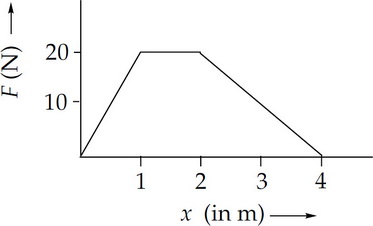A particle of mass m = 1.0 kg is acted upon by a variable force, F(x), whose strength is given by the graph given above. If the particle's speed was zero at x = 0, what is its speed at x = 4 m?

2. The radius of a collapsing spinning star (assumed to be a uniform sphere) decreases to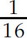its initial value. What is the ratio of the final rotational kinetic energy to the initial rotational kinetic energy?

3.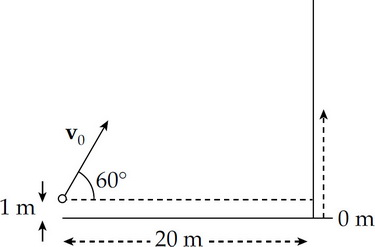A ball is projected with an initial velocity of magnitude v0 = 40 m/s toward a vertical wall as shown in the figure above. How long does the ball take to reach the wall?

4. If L, M, and T denote the dimensions of length, mass, and time, respectively, what are the dimensions of impulse?

5.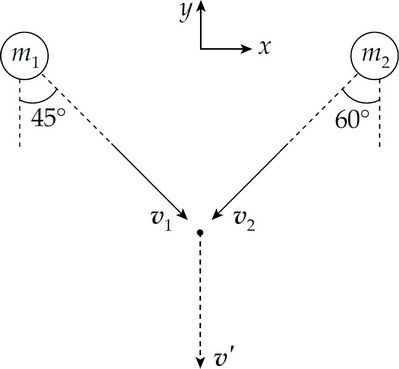The figure shown is a view from above of two clay balls moving toward each other on a frictionless surface. They collide perfectly inelastically at the indicated point and are observed to then move in the direction indicated by the post-collision velocity vector, v'. If m1 = 2m2 what is v2?

6.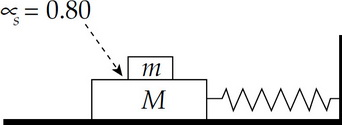In the figure above, the coefficient of static friction between the two blocks is 0.80. If the blocks oscillate with a frequency of 2.0 Hz, what is the maximum amplitude of the oscillations if the small block is not to slip on the large block?

7. When two objects collide, the ratio of the relative speed after the collision to the relative speed before the collision is called the coefficient of restitution, e. If a ball is dropped from height H1 onto a stationary floor, and the ball rebounds to height H2, what is the coefficient of restitution of the collision?

8.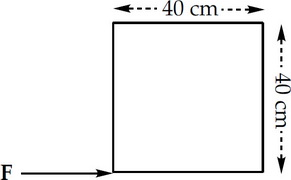The figure above shows a square metal plate of side length 40 cm and uniform density, lying flat on a table. A force F of magnitude 10 N is applied at one of the corners, as shown. Determine the torque produced by F relative to the center of rotation.

9.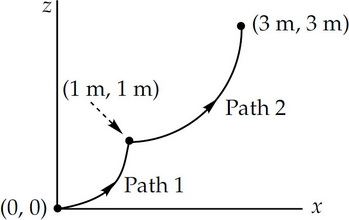A small block of mass m = 2.0 kg is pushed from the initial point (xi, zi) = (0 m, 0 m) upward to the final point (xf, zf) = (3 m, 3 m) along the path indicated. Path 1 is a portion of the parabola z = x2, and Path 2 is a quarter circle whose equation is (x – 2)2 + (z – 2)2 = 2. How much work is done by gravity during this displacement?

10.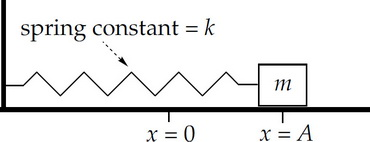In the figure shown, the block (mass = m) is at rest at x = A. As it moves back toward the wall due to the force exerted by the stretched spring, it is also acted upon by a frictional force whose strength is given by the expression bx, where b is a positive constant. What is the block's speed when it first passes through the equilibrium position (x = 0)?